# Team:SBS SH 112144/Model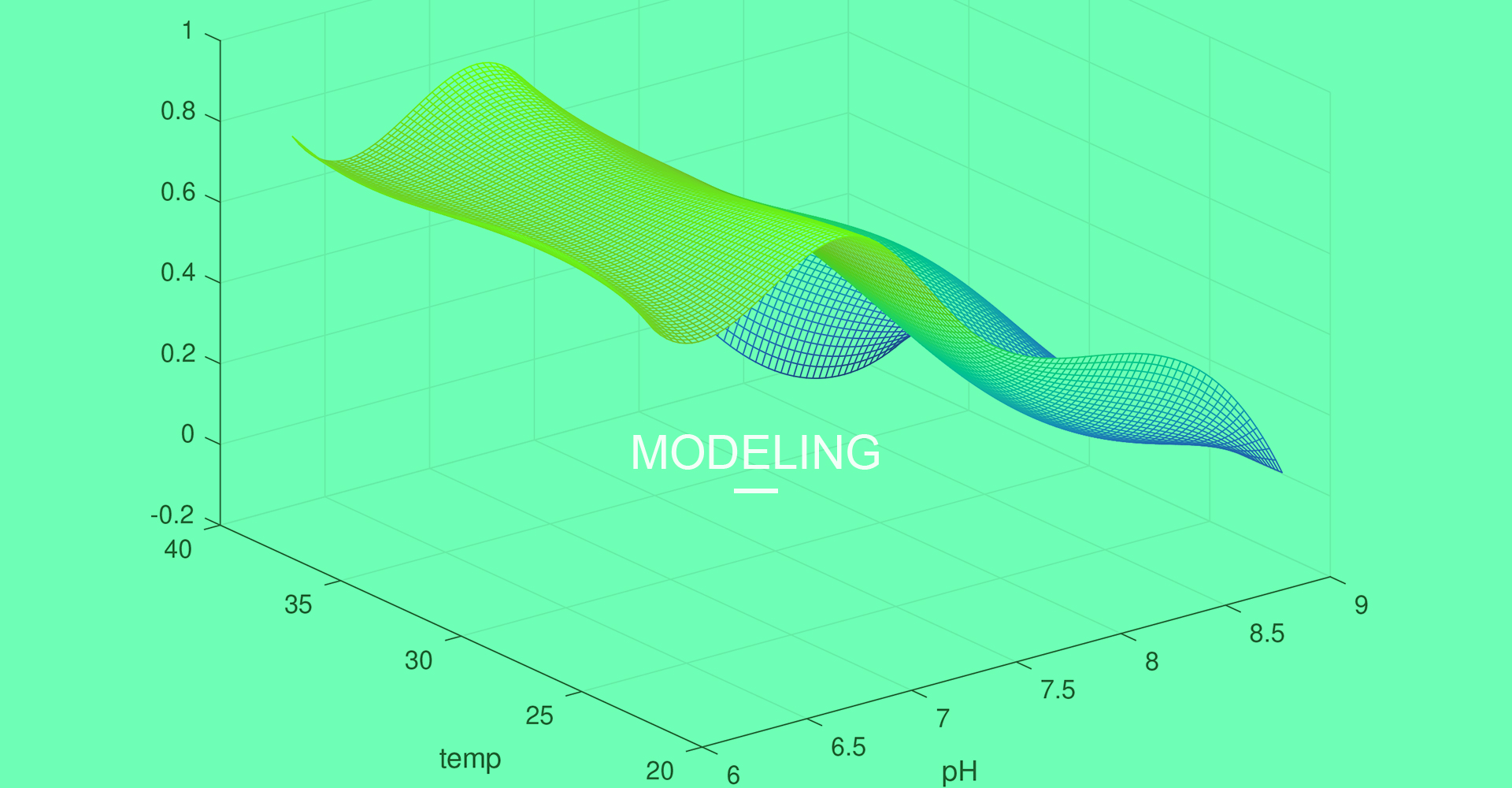#### Overview

Modeling, which involve rigorous data processing and complex mathematical computation, is often crucial for the discoveries in the realm of science. Our models mainly provide a solid foundation upon which we could design our device and build its prototype. Through background research, we have discovered four significant variables for the successful operation of the device and its potential commercial use. Therefore, we developed mathematical models to explore the optimal combination of four variables and to guide the experiments in wet lab.

One key problem in our wet lab is the difficulty precise data. How we give the data enough validity to support the work afterwards is worth discussing about. Another difficulty lies in the complexity of the optimal combination of four dimensional variables. Will we be able to obtain this combination to satisfy our needs? Our models gave a positive answer!

#### Model

##### A. Intro to variables

To achieve our goal of optimizing the setting of our device, we need to determine the parameters we are interested in. According to some background research, we plan to focus on pH, temperature, protein concentration and reaction time.

1. pH and temperature: because enzyme's catalytic function is hugely dependent on the pH and temperature of the surrounding environment, and even a slight deviation from the optimal parameter might denature some of the enzyme, we wish to figure out the optimal pH and temperature in the reaction that could best "bring out" the lysozyme's potential.

2. Protein concentration: the production and purification of this protein require a decent cost of material and manpower. Plus, methods to immobilize enzymes such as entrapment and cross-linkage are not easy to achieve. It would be cost-effective to identify the appropriate concentration of the enzyme required for the reaction thus prevent waste.

3. Reaction time: the concern about time is due to two reasons. First of all, time is directly related to efficiency of the facility, and a commercial company always prefer the more efficient option. Most importantly, since the end goal of our device is to be able to provide the different metabolites and component of cyanobacteria for medical/ scientific research, biofuel manufacturing and agricultural production, we would like to maintain the activity and completeness of those parts as intact as possible. Our device the cyanobacteria is in a dynamic state, thus overtime reaction might cause damage to crucial components such as chlorophylls, and not to mention all those chemicals such as bugbuster mixed in the reaction system. To summarize, we want the reaction time to be both enough for the cleavage and minimizing the damage to the cyanobacterial components.

##### B. Optimized Sparse Matrix

In the field of quantitative biology, free-form surfaces need to be constructed from discretely observed shape points. Since the properties of biological data are difficult to express with a mathematical function, it is necessary to first establish a regular grid and then perform interpolation calculation on the basis of the grid.

Our model implements two-dimensional spline interpolation of the Green function around the nearest neighbor points of the interpolation point[2,3]. By doing this we could reduce the calculation time; by using the nearest neighbors around the interpolation point to move the interpolation, changing the equilibrium of the distribution of the value points sequentially helps to improve the stability of the interpolation results.

As we know, the surface s(x) can be expressed as:

$$Function 1: s(x) = T(x) + \sum w_jg(x,x_j)$$

In equation, the weight w is satisfied:

$$Function 2: s(x) = \sum w_jg(x_i,x_j), i=1,2,...,n.$$

The Green function g(xi,xj) is expressed as:

$$Function 3: g(x_i,x_j)=|x_i,x_j|^2[In(|x_i,x_j|)-1]$$

In our model, the point to be interpolated is taken as the center, and the radius of variation is such that the number of known points falling into the circle is equal to the threshold k. The moving Green's base function spline two-dimensional interpolation algorithm is as follows:

1)If there are n known points, the interpolation point is $P_0(x_0,y_0)$, the nearest neighbors participating in the P point interpolation calculation are k, and the smaller k value (such as k < 30) will affect the calculation accuracy; increasing the k value can improve the interpolation accuracy, but When a certain threshold is reached (usu- ally less than 100), saturation occurs, that is, the interpolation accuracy is no longer improved. Calculate the distance $r_0$ from $P_0(x_0,y_0)$ and known points$(x_i,y_i)$:

$$Function 4: r_{0i} =\sqrt{(x_i-x_0)^2+(y_i-y_0)^2},i=1,2,...,n$$

2) Let the matrix that included k known points be $X=[x_1 x_2...x_k]^T, Y=[y_1 y_2...y_k]^T, Z=[z_1 z_2...z_k]^T$. Let the k-order square matrix G be

$$\left[ \begin{matrix} d_{11}& d_{12} & \cdots & d_{1k} \\ d_{21}& d_{22} & \cdots & d_{2k} \\ \vdots & \vdots & \ddots & \vdots \\ d_{k1}& d_{k2} & \cdots & d_{kk} \\ \end{matrix} \right]$$

so the $k\times 1$ weight matrix:

$$Function 5: W=G^{-1}Z$$

3) $1\times k$ matrix G of the interpolation point $p_0(x_0, y_0)$ is calculated :

$$Function 6: G_p = [d_{01} d_{02} ... d_{0k}]$$

Using the corresponding result calculated in step 1), the interpolated value Z of the $p_0$ point is calculated as:

$$Function 7: z_p = G_pW$$

4)Repeat steps 1) to 3) to find other points' interpolation in sequence.

As shown in Fig.1 and Fig.2 below. We use this model to optimize the interpolation of our data.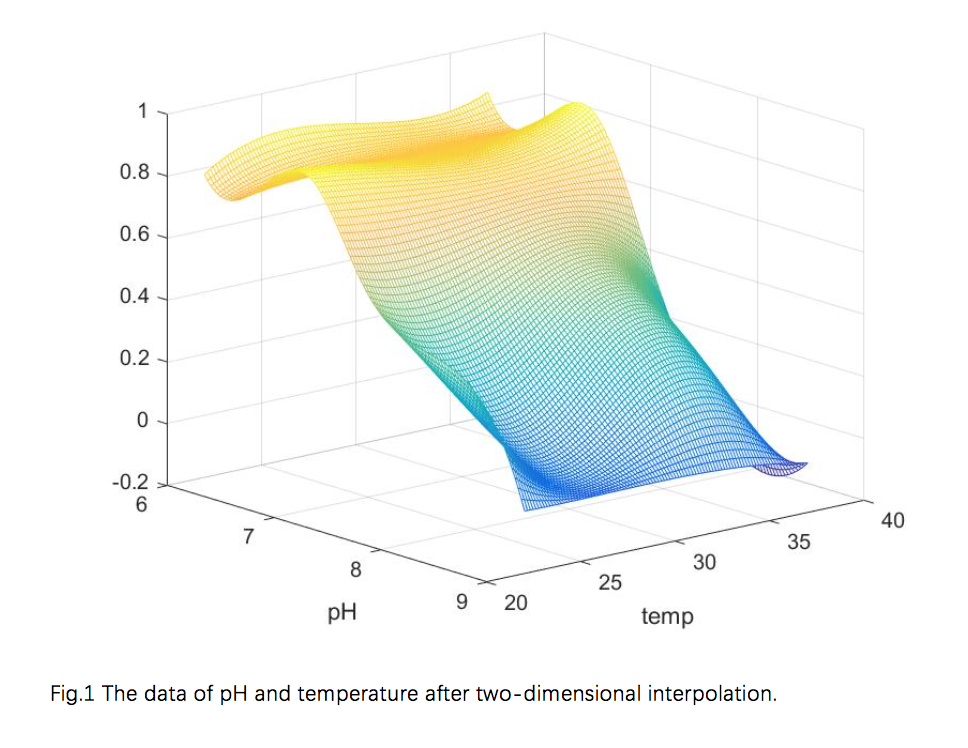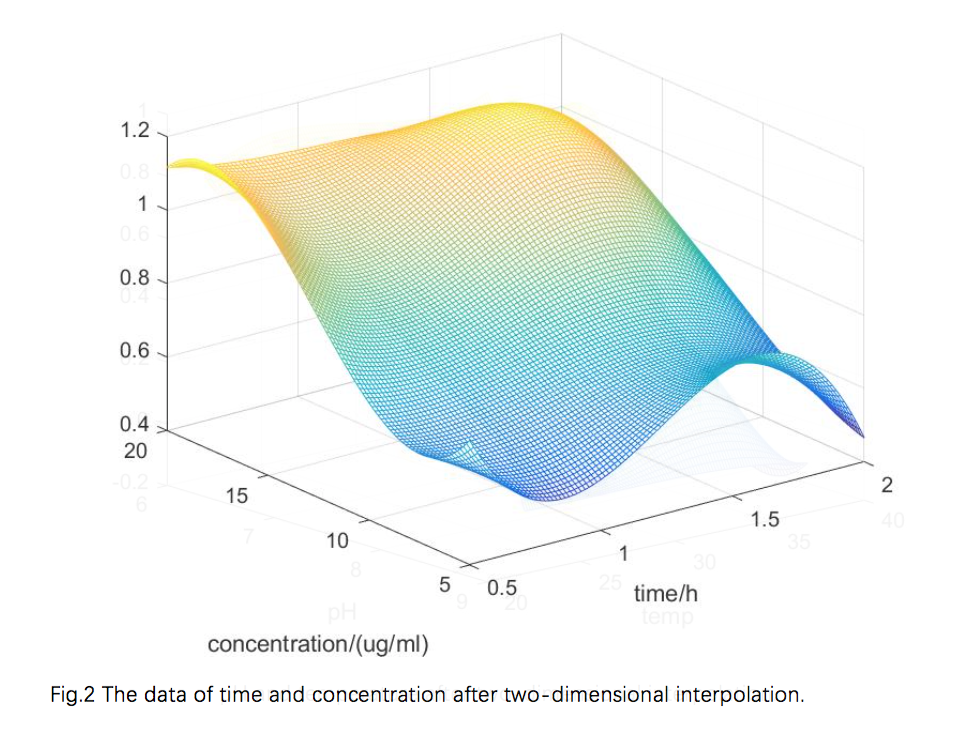##### C. Using Particle Swarm Optimization Algorithm to Find Global Optimal Solution

Solving the optimal solution problem is like climbing a mountain, it is easy to find the highest point of a mountain in a simple environment. However, in terms of complex environment, the main difficulty encountered by problem solvers searching such environments is the presence of several local optima (peaks) from which it is difficult to find better solutions. Such difficulty is illustrated by figure 3: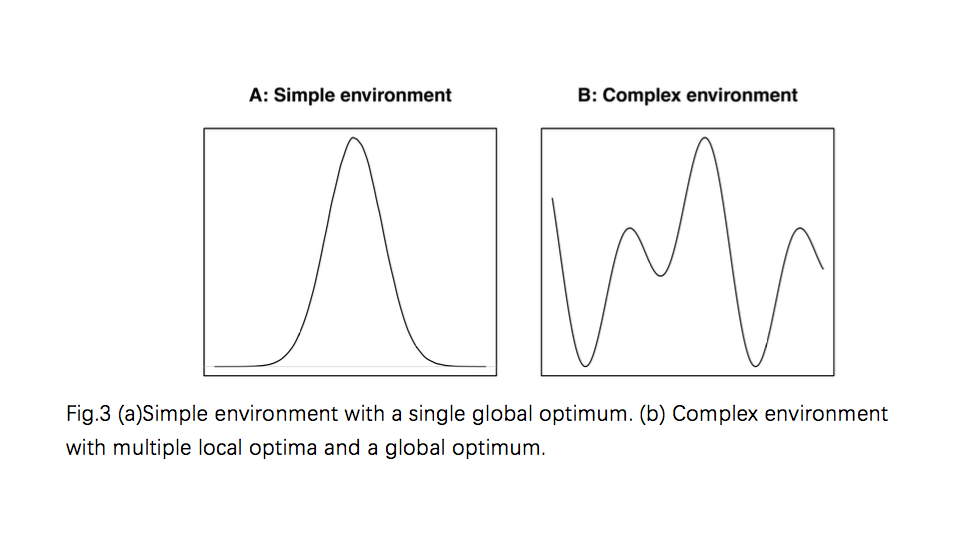The particle swarm optimization algorithm (PSO)[4,5] is derived from the study of the foraging behavior of the birds. The researchers found that the birds often suddenly change direction, spread, and bearing during the flight, and their behavior is unpredictable, but the overall pattern is consistent. They also maintain the most suitable distance between individuals. Through the study of the behavior of similar biological groups, it is found that there is an information sharing mechanism within each biological group, which provides an advantage for the evolution of the group, which is also the basis of PSO algorithm.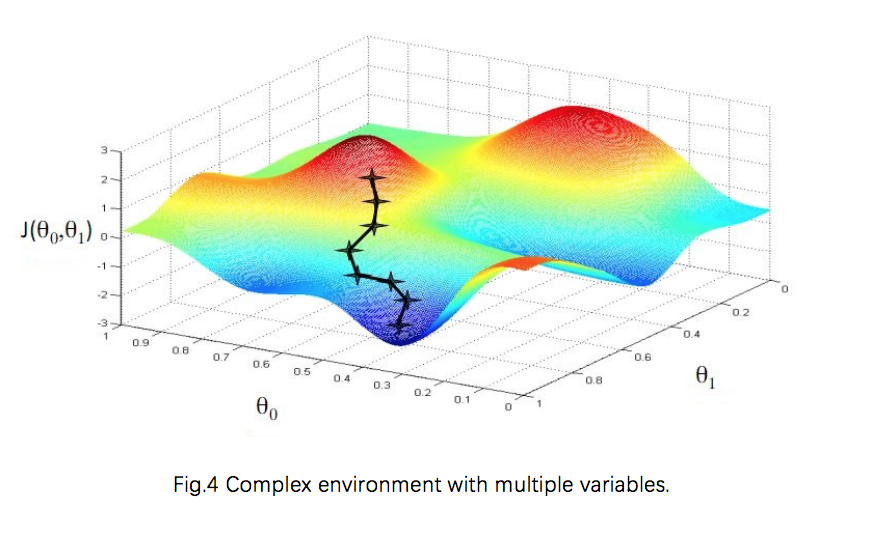Particle i in PSO algorithm will change its speed and position according to the formula below

$$Function 8: \begin{split} V_i = &w\times V_i + c_1\times rand()\times \\ &(pBest[i]-X_i)+c_2\times Rand()\times(pBest[g]-X_i),\\ Xi=&Xi+V_i, \end{split}$$

pBest represents individual extremum. The formula consists three parts: the first is the particle's previous speed, indicating the current state of the particle; the second is the cognitive model, which means the particle itself; the third part is the social model. Three parts together determine the spatial search capabilities of the particle. Part 1 provides the ability to balance global and local searches. Part 2 gives the particle a strong global search capability, avoiding local minima. Part 3 shows information shared between particles. Under the combined effect of these three parts, the particles can effectively reach the optimize position.

We use the PSO algorithm to find the optimal solution for the four variables, just indicated by Fig.5.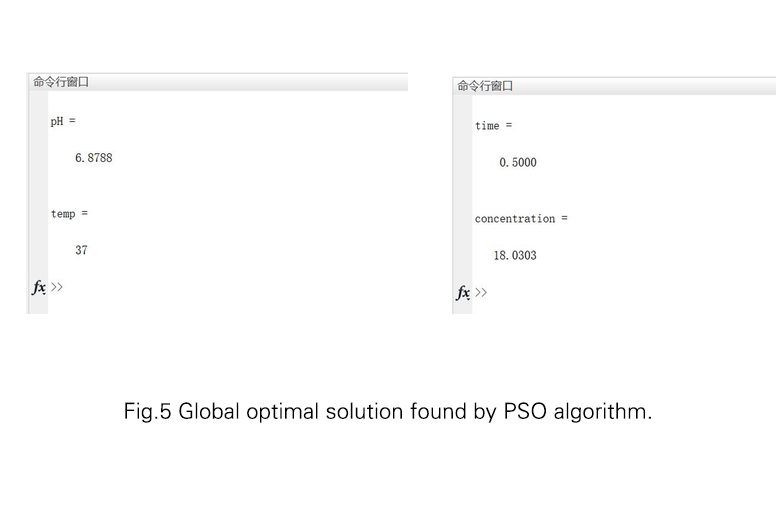#### References

1. Mehta, K. K., Evitt, N. H. & Swartz, J. R. Chemical lysis of cyanobacteria. Journal of Biological Engineering 9, (2015).

2. Wessel, Paul, and D. Bercovici. "Interpolation with Splines in Tension: A Green's Function Approach." Mathematical Geology 30.1(1998):77-93.

3. Smith, W. H. F., and P. Wessel. "Gridding with continuous curvature splines in tension." Geophysics 55.3(1990):293-305.

4. Eberhart, R., and J. Kennedy. "A new optimizer using particle swarm theory." MHS'95. Proceedings of the Sixth International Symposium on Micro Machine and Human Science IEEE, 2002:39-43.

5. Zhao, Bo, and Y. J. Cao. "Multiple objective particle swarm optimization technique for economic load dispatch." Journal of Zhejiang University-SCIENCE A 6.5(2005):420-427.